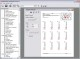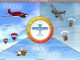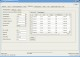Mathematics Worksheet Factory 3.1 A powerful, easy-to-use math worksheet generation program. SharewareLabMathX 1.0 LabMathX is a program for advanced mathematics, data display, and data archiving. SharewareEasy Calculat X 2.0.1 Easy Calculat is a calculator that can do simple calculations such and slightly more advanced mathematics like trigonometry. FreewareMath Flight 2.3 Fly High while having fun learning basic mathematics with Math Flight! SharewareMath-Flight 2 2.3 Fly High while having fun learning basic mathematics with Math Flight! SharewareZinsMath 4.01 financial mathematics SharewareMath Resource Studio 4.4.2 A powerful, easy-to-use math worksheet generation program. DemoMathDoggerX 0.0.1 MathDoggerX is a very very very simple Mathematics tutor. FreewareMathProf 4.0 MathProf is an easy to use mathematics program within approximately 180 subrouti SharewareWorkSheet Maker 2.3.3 WorkSheet Maker is a teaching tool for mathematics. Freeware